Next: Ladders and walls Up: Statics Previous: Equilibrium of a laminar

## Rods and cables

Consider a uniform rod of mass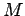and length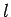which is suspended horizontally via two vertical cables. Let the points of attachment of the two cables be located distances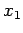and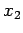from one of the ends of the rod, labeled. It is assumed that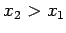. See Fig. 91. What are the tensions,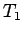and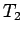, in the cables?

Let us first locate the centre of mass of the rod, which is situated at the rod's mid-point, a distance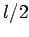from reference point(see Fig. 91). There are three forces acting on the rod: the gravitational force,, and the two tension forces,and. Each of these forces is directed vertically. Thus, the condition that zero net force acts on the system reduces to the condition that the net vertical force is zero, which yields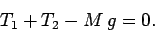(470)

Consider the torques exerted by the three above-mentioned forces about point. Each of these torques attempts to twist the rod about an axis perpendicular to the plane of the diagram. Hence, the condition that zero net torque acts on the system reduces to the condition that the net torque at point, about an axis perpendicular to the plane of the diagram, is zero. The contribution of each force to this torque is simply the product of the magnitude of the force and the length of the associated lever arm. In each case, the length of the lever arm is equivalent to the distance of the point of action of the force from, measured along the length of the rod. Hence, setting the net torque to zero, we obtain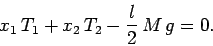(471)

Note that the torque associated with the gravitational force,, has a minus sign in front, because this torque obviously attempts to twist the rod in the opposite direction to the torques associated with the tensions in the cables.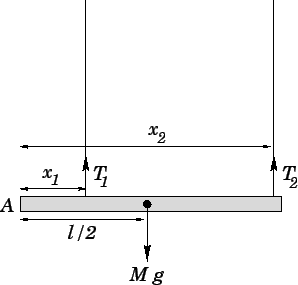The previous two equations can be solved to give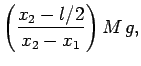(472)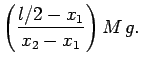(473)

Recall that tensions in flexible cables can never be negative, since this would imply that the cables in question were being compressed. Of course, when cables are compressed they simply collapse. It is clear, from the above expressions, that in order for the tensionsandto remain positive (given that), the following conditions must be satisfied: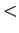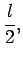(474)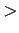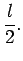(475)

In other words, the attachment points of the two cables must straddle the centre of mass of the rod.

Consider a uniform rod of massand lengthwhich is free to rotate in the vertical plane about a fixed pivot attached to one of its ends. The other end of the rod is attached to a fixed cable. We can imagine that both the pivot and the cable are anchored in the same vertical wall. See Fig. 92. Suppose that the rod is level, and that the cable subtends an anglewith the horizontal. Assuming that the rod is in equilibrium, what is the magnitude of the tension,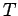, in the cable, and what is the direction and magnitude of the reaction,, at the pivot?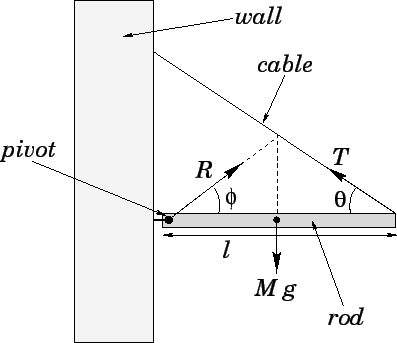As usual, the centre of mass of the rod lies at its mid-point. There are three forces acting on the rod: the reaction,; the weight,; and the tension,. The reaction acts at the pivot. Let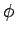be the angle subtended by the reaction with the horizontal, as shown in Fig. 92. The weight acts at the centre of mass of the rod, and is directed vertically downwards. Finally, the tension acts at the end of the rod, and is directed along the cable.

Resolving horizontally, and setting the net horizontal force acting on the rod to zero, we obtain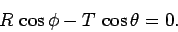(476)

Likewise, resolving vertically, and setting the net vertical force acting on the rod to zero, we obtain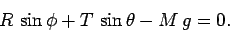(477)

The above constraints are sufficient to ensure that zero net force acts on the rod.

Let us evaluate the net torque acting at the pivot point (about an axis perpendicular to the plane of the diagram). The reaction,, does not contribute to this torque, since it acts at the pivot point. The length of the lever arm associated with the weight,, is. Simple trigonometry reveals that the length of the lever arm associated with the tension,, is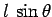. Hence, setting the net torque about the pivot point to zero, we obtain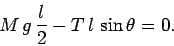(478)

Note that there is a minus sign in front of the second torque, since this torque clearly attempts to twist the rod in the opposite sense to the first.

Equations (476) and (477) can be solved to give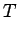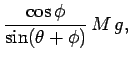(479)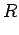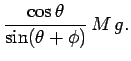(480)

Substituting Eq. (479) into Eq. (478), we obtain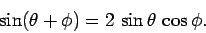(481)

The physical solution of this equation is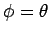(recall that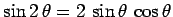), which determines the direction of the reaction at the pivot. Finally, Eqs. (479) and (480) yield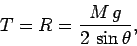(482)

which determines both the magnitude of the tension in the cable and that of the reaction at the pivot.

One important point to note about the above solution is that ifthen the lines of action of the three forces--,, and--intersect at the same point, as shown in Fig. 92. This is an illustration of a general rule. Namely, whenever a rigid body is in equilibrium under the action of three forces, then these forces are either mutually parallel, as shown in Fig. 91, or their lines of action pass through the same point, as shown in Fig. 92.Next: Ladders and walls Up: Statics Previous: Equilibrium of a laminar
Richard Fitzpatrick 2006-02-02